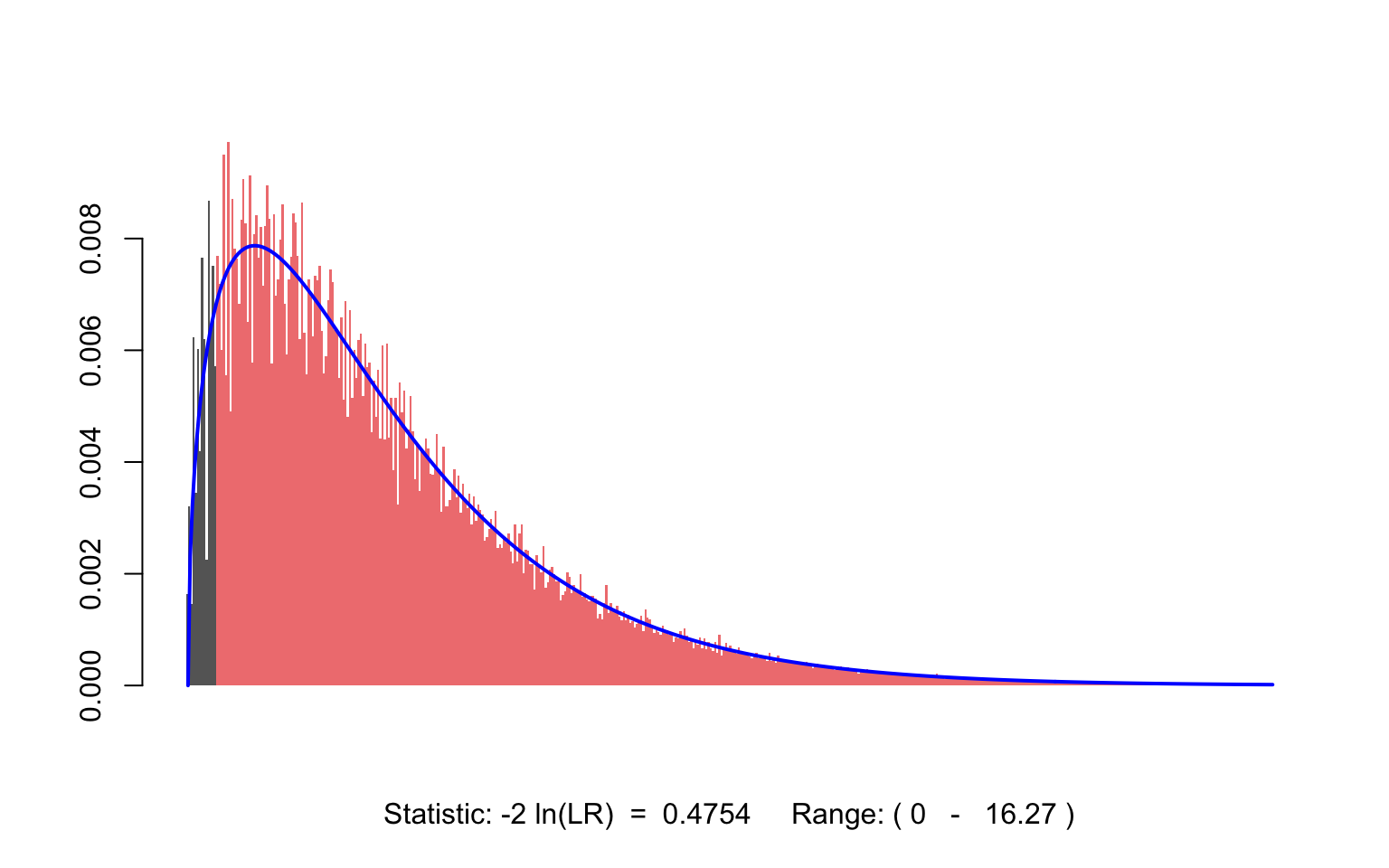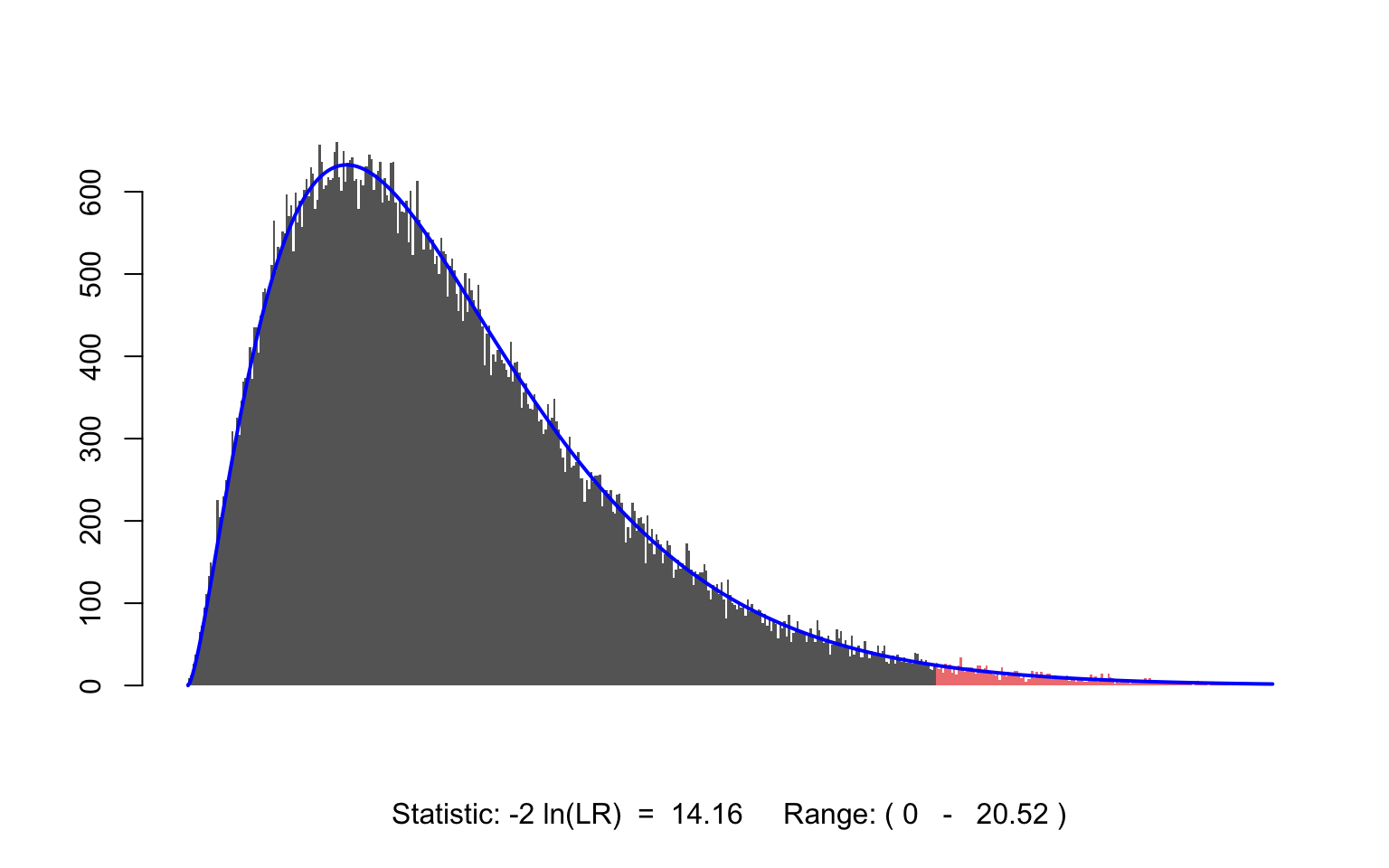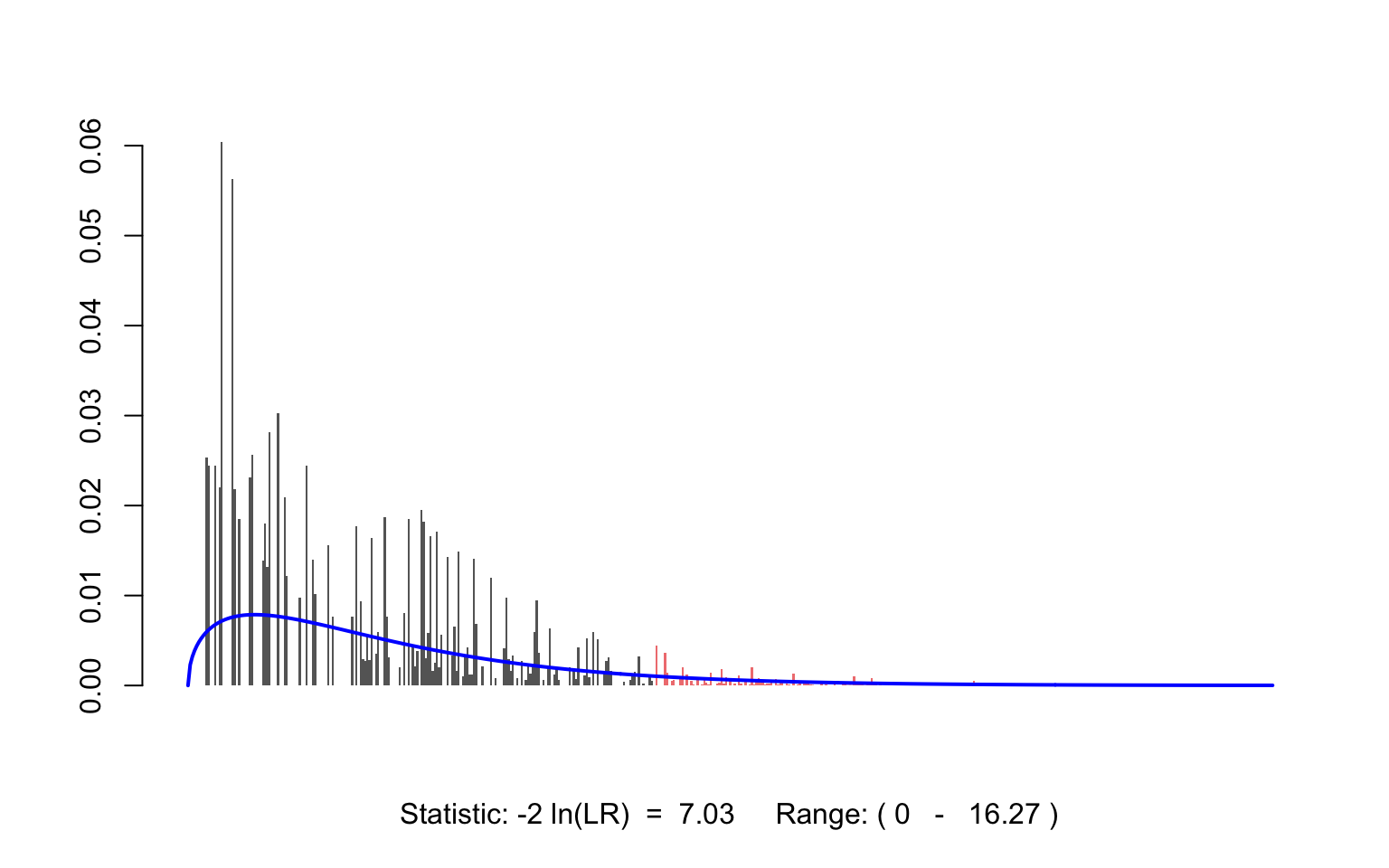# XNomial – Exact Test For Multinomial

### Contents

• Purpose – 1
• What’s in the package? – 2
• The goodness of exact tests – 3
• Comparing the goodness of fit – 4
• xmulti() versus xmonte()5
• Example 1: Mendel’s data – 6
• Example 2: Mendel’s (hypothetical) data – 7
• Example 3: Multinomial with Poisson probabilities – 8

### Purpose

Suppose you observed $$n$$ independent objects, each of which is one of $$k$$ types. You hypothesize a set of probabilities corresponding to these types, and wish to test how well the observed set fits. The key assumptions making this a multinomial hypothesis are: * Each object is an independent trial * The same set of probabilities apply to all

The question is this: if you were to repeat the experiment many times, what is the probability that the data would deviate from your hypothesis at least as much as the observed results assuming your hypothesis is true. This probability is, of course, the $$P$$ value which may be computed by functions in XNomial.

### What’s in the package?

There are two functions: xmulti() and xmonte(). They are both aimed at finding the $$P$$ value used to test the goodness-of-fit for a multinomial distribution with fixed probabilities. Although these two functions do much the same thing, they use very different approaches: * With xmulti(), all possible outcomes will be examined for an exact test * With xmonte(), a random set of possible outcomes is used for a monte-carlo test

### The goodness of exact tests

Why bother with an exact test anyway? Aren’t there perfectly good asymptotic tests like the $${\chi ^2}$$ available for these situations that provide reasonably good approximations to the true $$P$$ value? The easy answer is that those approximations aren’t always good enough, and it can be hard to tell just how accurate they are for a given case. A more subtle answer, however, is that exact tests are easier for non-statisticians to understand. In more than 30 years of trying to explain statistical reasoning to my fellow biologists I’ve often found that my listener’s eyes start to glaze over when I talk about limiting distributions for large samples. On the other hand, if I can simply say that I examined all possible outcomes of the experiment to determine how “unlikely” the observed one is, that’s something they can usually relate to. Similarly, I find that Monte Carlo tests are just as easy to explain. Most scientifically-minded people, including the statistics-averse, can readily understand the concept of trying a large number of random cases to get an idea of how unlikely a particular outcome would be. In general, statistical procedures are of little real value unless they can communicate something meaningful to the your audience, and I find that exact tests do this job admirably!

### Comparing the goodness of fit

It is not always clear what is the best way to compare possible outcomes with the actual observed results in order to determine which is a better fit to the model. There are actually three commonly used methods for multinomial data:

1. The likelihood ratio: This is the ratio of the probability of the observed result under the null hypothesis over its probability given the alternative. We’ll assume there is no specific alternative in mind and just use the best-fitting multinomial distribution for the outcome being considered. It’s convenient to work with log of this quantity (the LLR) given by: $latex LLR = \sum\limits_{i = 1}^k {{m_i}\ln \left( {\frac{{n{p_i}}}{{{m_i}}}} \right)}$ where $${{m_i}}$$ is the number of objects in category $$i$$ and $$p_i$$ is the hypothesized probability of that category.
2. The multinomial probability itself: This is simply the probability of a given outcome under the null: $latex {\mathop{\rm P}\nolimits} \left( {{m_1},{m_2}, \ldots ,{m_k}} \right) = \frac{{n!}}{{{m_1}!{m_2}! \cdots {m_k}!}}p_1^{{m_1}}p_2^{{m_2}} \cdots p_k^{{m_k}}$
3. The classic chi-squared statistic (Pearson’s $$X ^2$$): $latex {X^2} = \sum\limits_{i = 1}^k {\frac{{{{\left( {{m_i} - n{p_i}} \right)}^2}}}{{n{p_i}}}}$

All three measures are commonly used, and which you choose is a matter of taste. It comes down to which one you think best matches your idea of what constitutes a good fit. Regardless of which of these measures you prefer, XNomial is equally useful for finding the associated $$P$$ value. In many cases, you will find similar $$P$$ values from all three measures. However, if you play around with XNomial a bit, you will quickly find cases where they differ substantially!

Personally, I have a clear preference for the LLR, and here are my reasons:

1. The ratio of probabilities seems like a straightforward way of comparing the null hypothesis with the best-fitting alternative.

2. Using the probability itself ignores the relative nature of the comparison. For example, suppose the observed outcome has a very low probability under the null hypothesis. That would seem to argue against the hypothesis, right? However, suppose the same outcome also has a very low probability under any of the alternative hypotheses considered. In that case, it simply means that something unusual has happened, and it does not argue either for or against the null hypothesis compared to the alternative(s).

3. The chi-squared statistic owes its popularity to the fact that it quickly approaches its asymptotic $${\chi ^2}$$ distribution which can be conveniently tabled in the back of statistics textbooks. With XNomial there is no need to consider the asymptotic distribution, and the $${X ^2}$$ statistic seems like more of a historical holdover.

Other measures are possible, and can be preferable in some circumstances. In particular, if the alternative hypotheses specify that the probabilities would deviate from the null hypothesis’ $$p_i$$ in a certain way, then a measure of goodness-of-fit can be tailored accordingly. The current version of XNomial only considers general alternative hypotheses.

### When to use xmulti() versus xmonte()

Simply put, xmulti is always preferable except when it takes too long. If it does, then you can get the same information from xmonte but with a bit less precision. Calling xmulti() generates all of the possible ways $$n$$ objects can be placed into $$k$$ categories, and computes the three measures of goodness-of-fit described above for each. The number of these combinations is $$latex {n+k-1 \choose k-1}$$. On my 2012 iMac, xmulti can handle about 10^8 combinations per second. If $$n$$ and $$k$$ are so large that xmulti takes too long, then it’s better to use xmonte which looks at only a random sampling of the combinations. Typically, a sample of 10^5 or 10^6 is adequate. This procedure is called a “Monte Carlo” test. There is no reason to prefer xmonte over xmulti unless the latter takes too long.

### Example 1: Gregor Mendel’s data

The Austrian monk performed some crosses with garden pea plants. In one experiment, he counted 556 seeds and classified them according to shape and color. The four categories were round/yellow, round/green, wrinkled/yellow and wrinkled/green, and his counts were 315, 108, 101 and 32 respectively.

peasF2 <- c(315, 108, 101, 32)
getwd()
##  "/private/var/folders/5v/rs7qt8vd68b93wgcg269066m0000gn/T/Rtmprb6saL/Rbuildf9a25b5a0a4/XNomial/vignettes"

According to his genetics model, these types should have appeared in the ratio of 9:3:3:1, so

peasExp <- c(9, 3, 3, 1)

How well did his data fit the hypothesis? We can test it with xmulti:

xmulti(peasF2, peasExp)
##
## P value (LLR) = 0.9261

Such a high $$P$$ value means Mendel’s data fit the model better than expected. (The tendency for Mendel’s results to fit too well has not escaped the attention of historians!)

We can ask for a more detailed report as follows:

xmulti(peasF2, peasExp, detail=3)
##
## P value  (LLR)  =  0.9261
## P value (Prob)  =  0.9382
## P value (Chisq) =  0.9272
##
## Observed:  315 108 101 32
## Expected ratio:  9 3 3 1
## Total number of tables:  28956759

Now it is clear that all three measures of goodness-of-fit give $$P$$ values in the same ballpark. This report also shows how many possible outcomes xmulti had to look at. Namely … $latex N ={{556 + 4 - 1} \choose {4-1}} = 28,956,759$ If we want to see the shape of the LLR’s distribution, we can ask xmulti to plot the histogram as follows:

xmulti(peasF2, peasExp, histobins=T)
##
## P value (LLR) = 0.9261The blue curve shows that the asymptotic $${\chi ^2}$$ distribution with 3 degrees of freedom is not a bad fit in this case.

### Example 2: Mendel’s (hypothetical) data

Now imagine that Mendel looked closer at his 556 seeds and noticed that the ones he had classified as yellow actually fell into two categories: light-yellow and dark-yellow. So now, instead of four kinds of seeds, he had six: namely …

1. round/dark-yellow
2. round/light-yellow
3. round/green
4. wrinkled/dark-yellow
5. wrinkled/light-yellow
6. wrinkled/green

… and the reclassified numbers might be:

rePeas <- c(230, 85, 108, 80, 21, 32)

in the same order. If we assume that the two shades of yellow represent peas that were homozygous (light-yellow) or heterozygous (dark-yellow) for the yellow allele, then from standard genetics we expect the ratios to be 6:3:3:2:1:1.

reExp <- c(6,3,3,2,1,1)

But when we try to test these results using xmulti we receive a warning that a full enumeration of all the possible ways to classify 556 seeds into 6 types is very large. In fact, $latex N ={{556 + 6 - 1} \choose {6-1}} = 454,852,770,372$ and it would take a computer like mine more than an hour to analyze them all. Therefore, a better option would be to use xmonte to look at just a random sample of the nearly half-a-trillion possible outcomes.

xmonte(rePeas, reExp)
##
## P value (LLR) = 0.01495 ± 0.0003838

Note that xmonte reports a standard error along with the estimated $$P$$ value. This is because we are only estimating $$P$$ based on a random sample. If you need a more accurate estimate, simply increase the parameter ntrials. As before, we can get a more detailed report and/or a histogram plot:

xmonte(rePeas, reExp, detail=3, histobins=T)
##
## P value (LLR) = 0.01495 ± 0.0003838
##  1e+05  random trials
##  Observed:  230 85 108 80 21 32
##  Expected Ratio:  6 3 3 2 1 1The red area of the histogram represents the $$P$$ value. In this case, $$P$$ is much smaller than in Mendel’s real data, indicating that the fit is not nearly so good, and we will want to modify our hypothesis. Perhaps the two shades of yellow were too close for the human eye to distinguish accurately and misclassifications occurred.

### Example 3: Multinomial with Poisson probabilities

Here is another example, also from genetics. Suppose you treat 100 chromosomes with a mutagen dose calibrated to deliver an average of 0.2 mutations per chromosome. You find that 84 of them received no mutations, 11 had exactly one mutation, 4 had exactly 2 mutations, and one chromosome had more than 2 but you could not count how many. So the observed counts are:

obsMut <- c(84, 11, 4, 1)

You wish to test whether these numbers are consistent with the number of mutations per chromosome being Poisson distributed with an average of 0.2 per chromosome.

To get the expected proportions in each category, use the Poisson distribution. Find the first three directly, then get the final one by subtraction:

probMut <- dpois(0:2, 0.2);
probMut <- 1 - sum(probMut); 

Now, test the hypothesis using xmulti

xmulti(obsMut, probMut, detail=3, histobins=T)
##
## P value  (LLR)  =  0.04799
## P value (Prob)  =  0.01991
## P value (Chisq) =  0.01875
##
## Observed:  84 11 4 1
## Expected ratio:  0.8187308 0.1637462 0.01637462 0.001148481
## Total number of tables:  176851The exact $$P$$ value of 0.048 is considerably smaller than the asymptotic value one would obtain, 0.071. By looking at the histogram we can see that this discrepancy is not unexpected given how different the actual distribution is compared with the blue curve.